# OzGrid

Lookup formulae reference sheet

Category: [Excel]  Demo Available

Lookup formulae reference sheet

 =INDEX() Returns the value of a cell in a table based on the column and row number. =INDEX(B3:B9,4) In the range B3:B9 provide the name of the 4th person.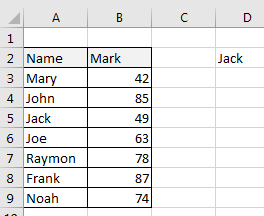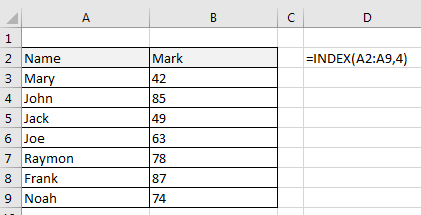=MATCH() Returns the position of a cell in a row or column. =MATCH(A5,A3:A9,2)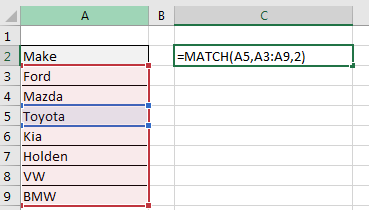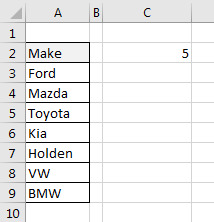=VLOOKUP A lookup function that searches vertically in a table. For example to find out what rate each staff member should be reimbursed for use of vehicle per km, use the VLOOKUP formula.   Look up the Make in the Reference table and when it is found use the value in column 2; use the word ‘FALSE’ at te end of the formula to provide an exact match.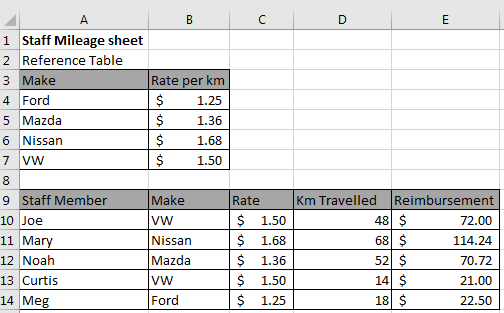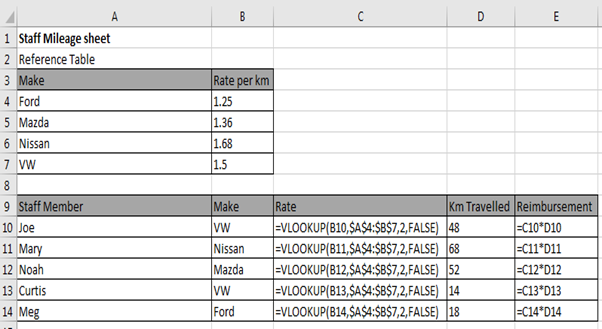=HLOOKUP A lookup function that searches vertically and horizontally in a table. Look up the model year as per C18 in the range B3:E18, and then put the model number as depicted by the 4th Row in the table.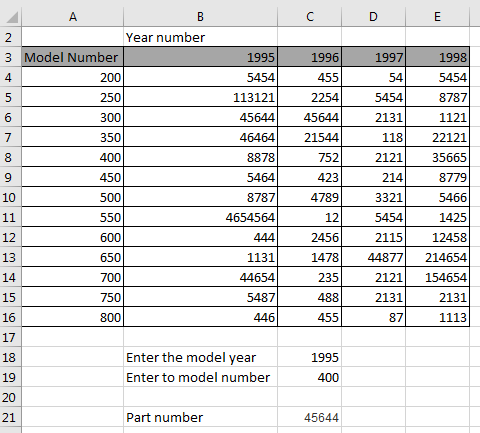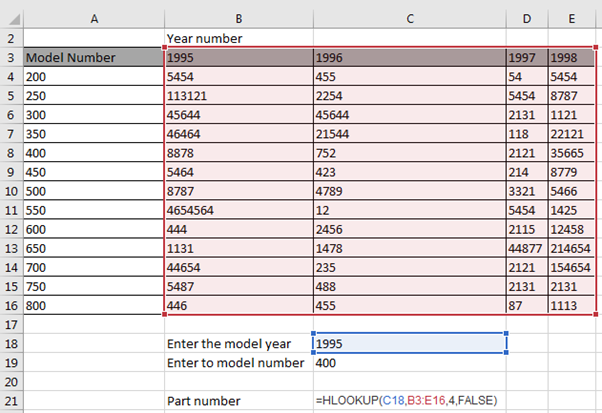=OFFSET Moves the reference of a cell by the number of rows and/or columns specified. The OFFSET function in Excel returns a cell or range of cells that is a specified number of rows and columns from a cell or range of cells. In the example below: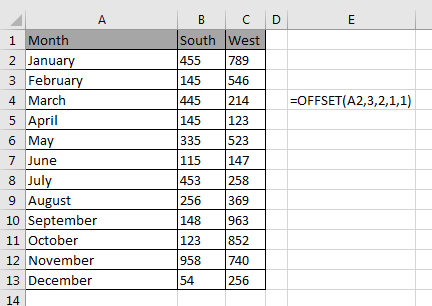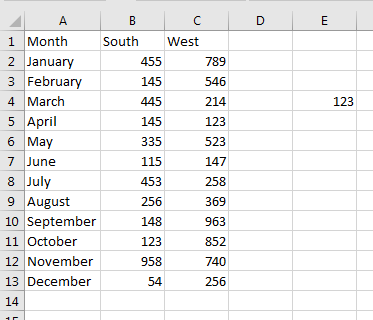Free Training Course: Lesson 1 - Excel Fundamentals

Microsoft ® and Microsoft Excel ® are registered trademarks of Microsoft Corporation. OzGrid is in no way associated with Microsoft.

Click here to visit our Free 24/7 Excel/VBA Help Forum where there are thousands of posts you can get information from, or you can join the Forum and post your own questions.

### Gallery

stars (0 Reviews)